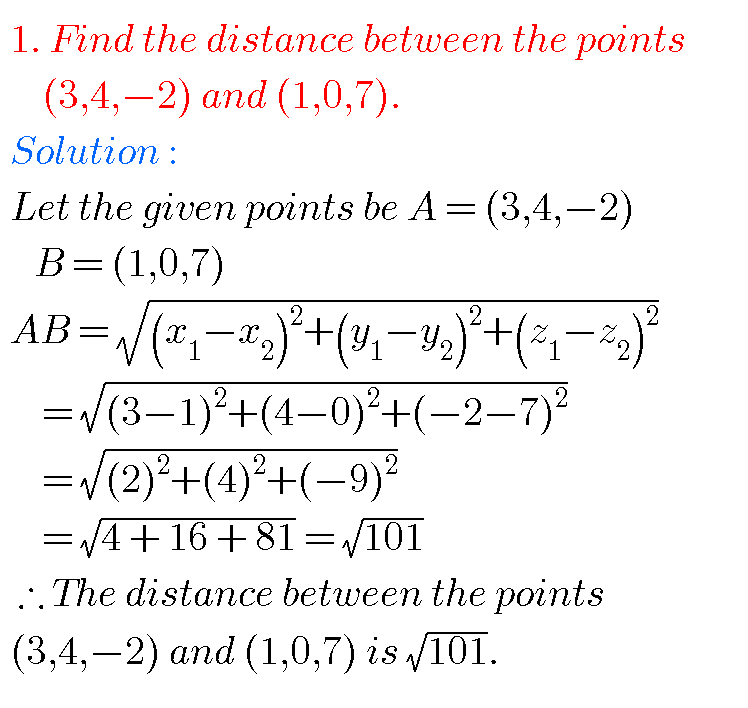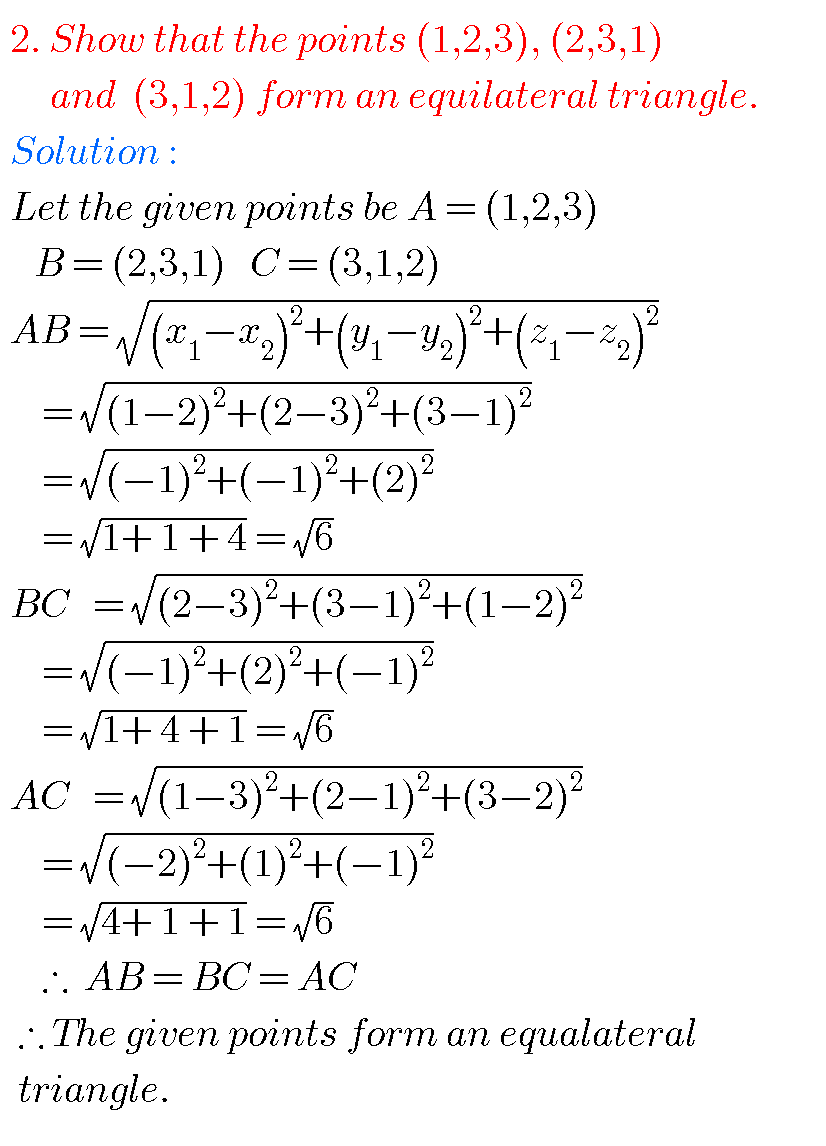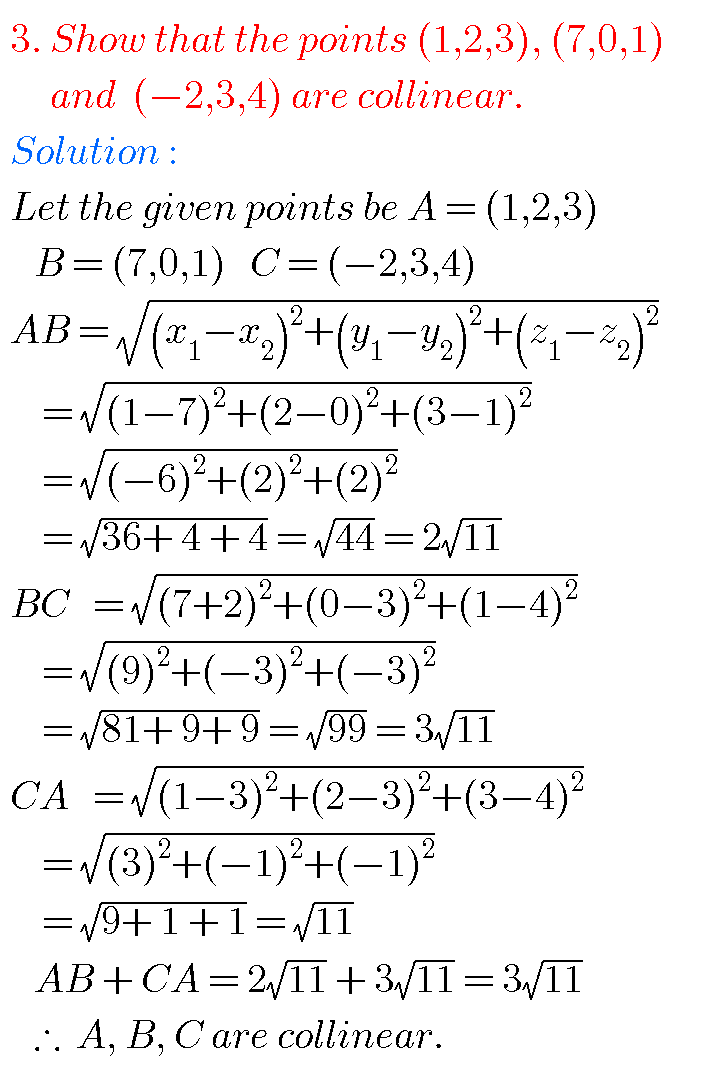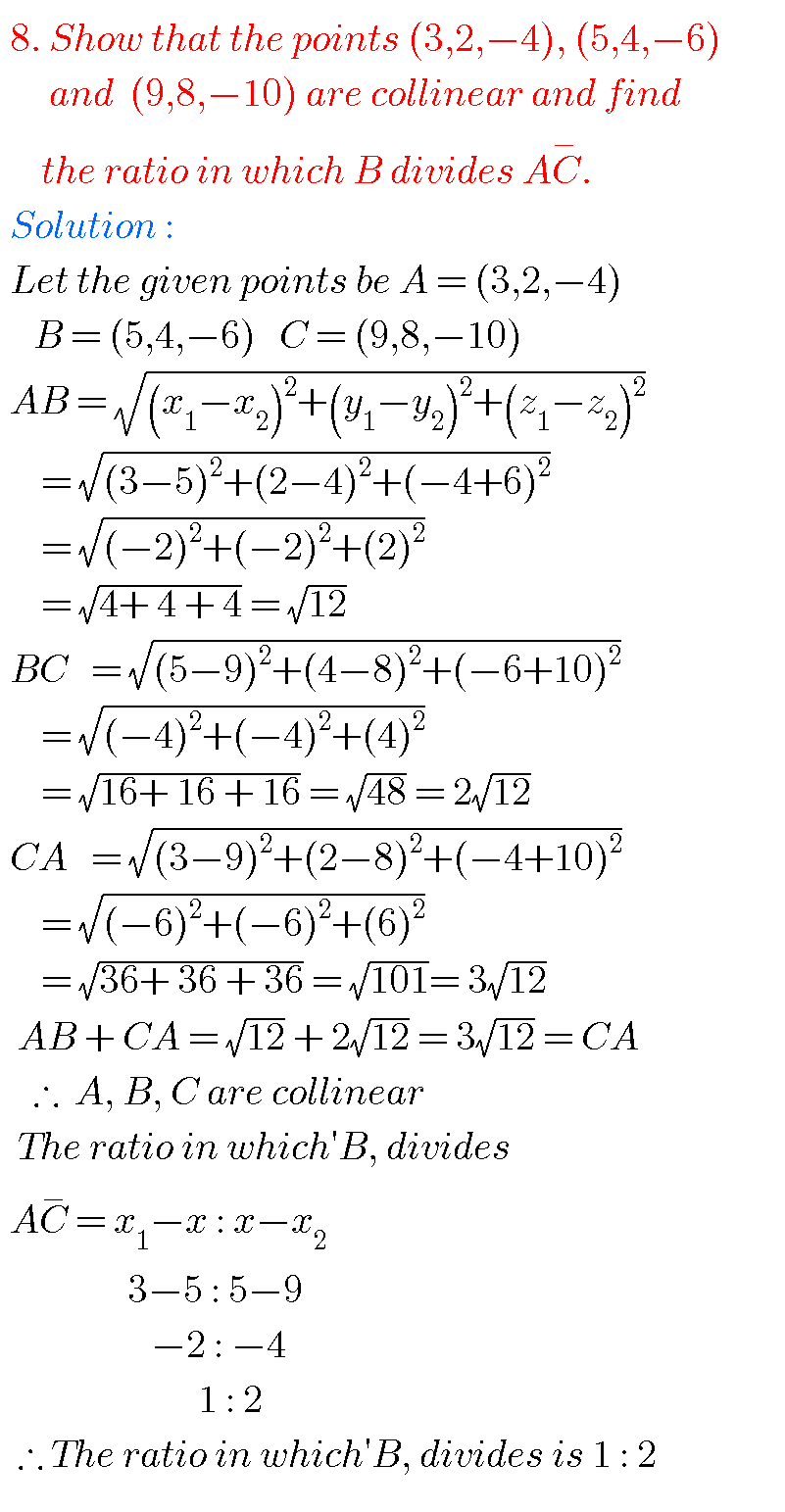THREE DIMENSIONAL COORDINATES INTERMEDIATE FIRST YEAR 1B CHAPTER 5 PROBLEMS WITH SOLUTIONS

Mathematics intermediate 1a and 1b solutions  for some problems are available here. These are very simple to understand.

Inter first year 1a : Functions, mathematical inductions, addition of vectors, product of vectors and trigonometry.

Inter first year 1b : Locus, transformation of axes, the straight line, pair of straight lines, three dimensional coordinates, direction cosines & ratios and applications of derivatives.

You can see the solutions for junior inter maths 1B

1Locus

Straight lines sa

Straight lines la

12. Rate measure

You can also see the solutions for junior inter maths 1A

3. Matrices

THREE DIMENSIONAL COORDINATES INTERMEDIATE FIRST YEAR 1B CHAPTER 5 PROBLEMS WITH SOLUTIONS

Junior inter maths chapter 5 three dimensional coordinates

Very short answer questionsNote : Observe the solutions and try them in your own methods.

Inter maths 1a solutions

Inter maths trigonometry solutions

Maths real numbers solutions class 10